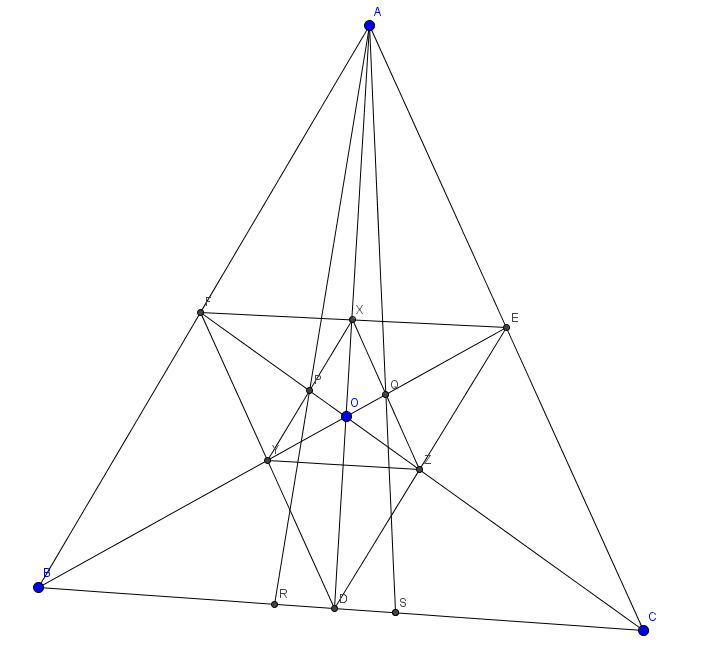# The Special Point in a Triangle 2

Geometry Level 5Let $O$ be a point in acute-angle triangle $ABC$.

$D$ is the intersection of $AO$ and $BC$. $E,F$ are defined similarly.

$X$ is the intersection of $EF$ and $AD$. $Y,Z$ are defined similarly.

Let $P$ be the intersection of $XY$ and $CF$ and $Q$ be the intersection of $XZ$ and $BE$.

$R$ is the intersection of $AP$ with $BC$ and $S$ is the intersection of $AQ$ with $BC$.

When $O$ is the circumcentre, $\frac{BR}{RC}=\frac{4 \sqrt{3}}{9}$.

When $O$ is the orthocentre, $\frac{BR}{RC}=\frac{2 \sqrt{3}}{3}$.

Find $\frac{4 \times \angle C}{\angle B}$ where we measure the angles in degrees.

Note: Where intersections between sides are described it is assumed the sides are extended if necessary.

The Special Point In A Triangle 1

×